Anzeige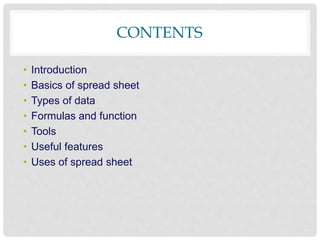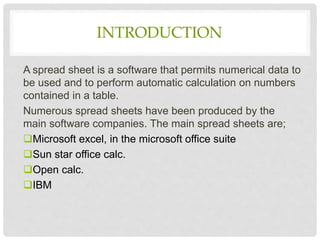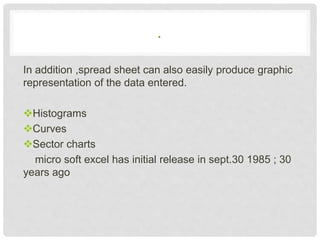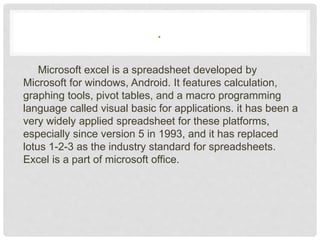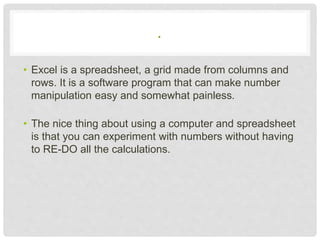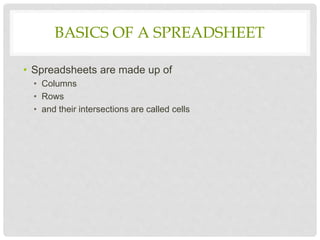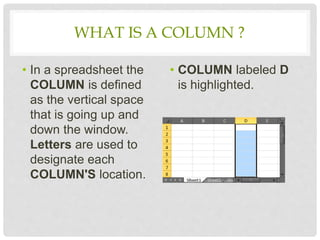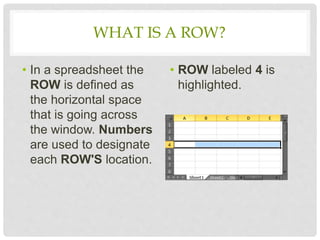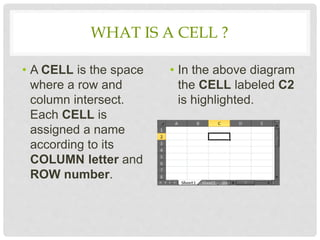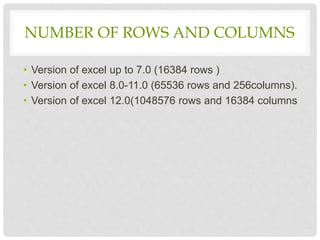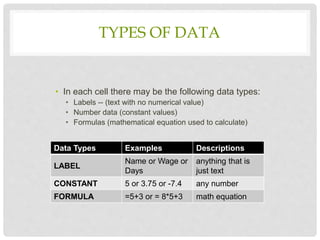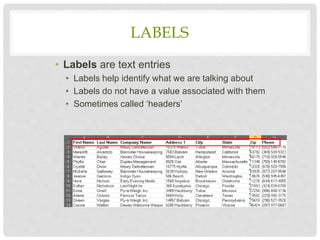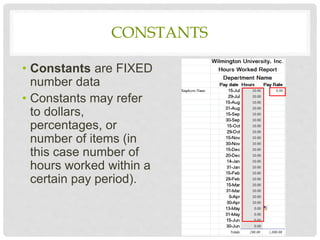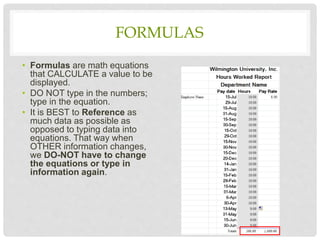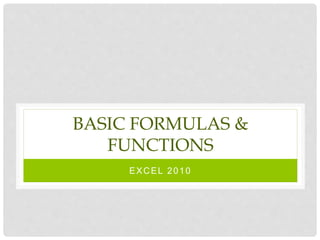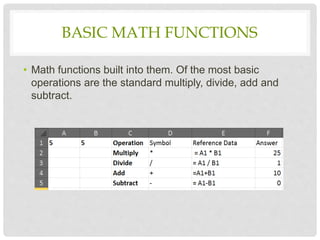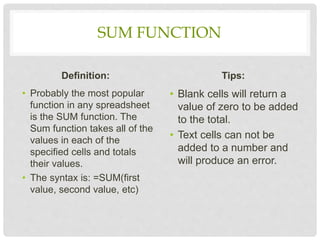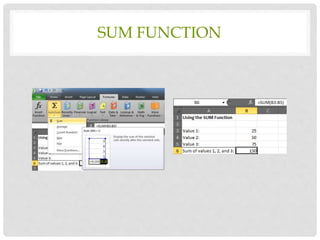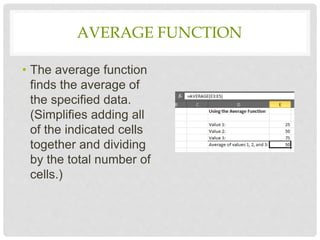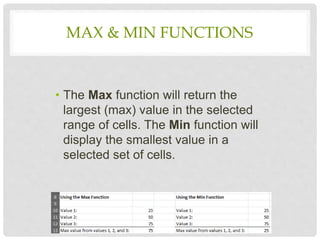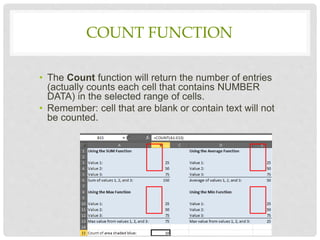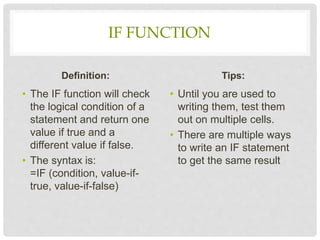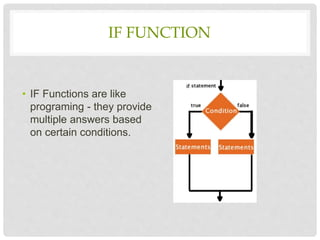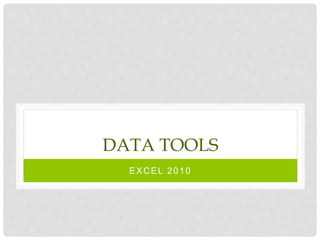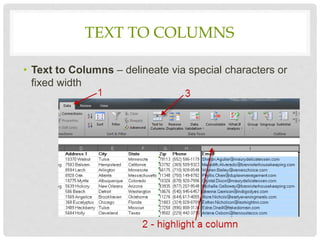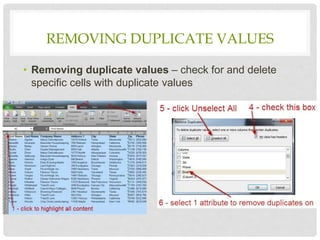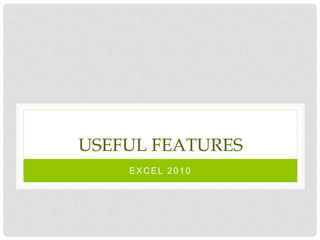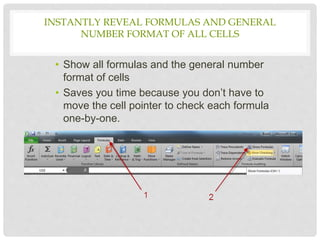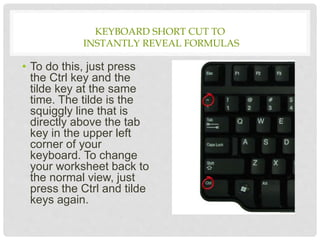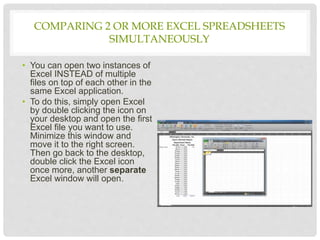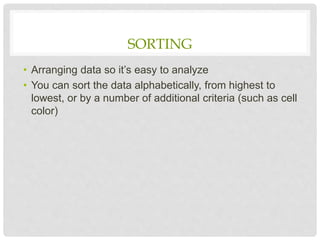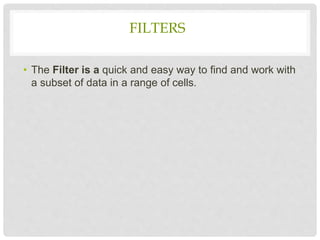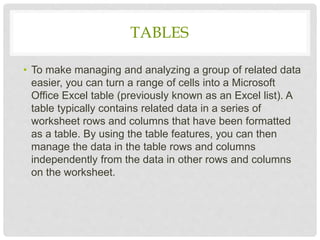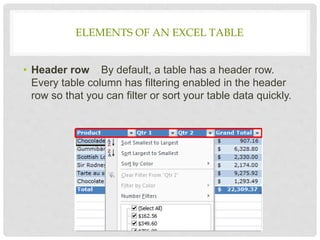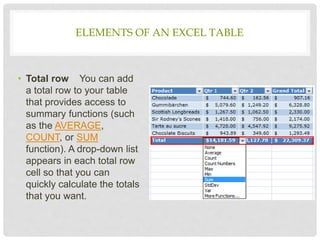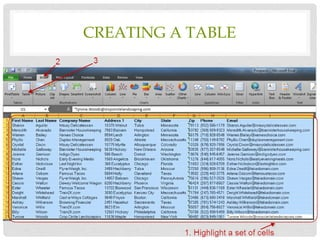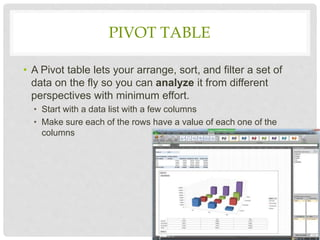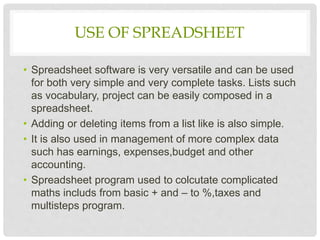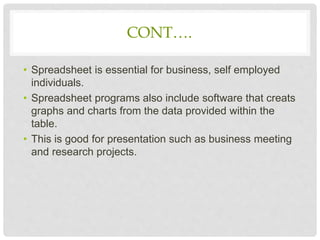1 von 41
Anzeige

1. UNIVERSITY OF MYSORE SUBJECT: Fundamentals of Information Technology SEMINOR ON : Spreadsheet presented by, Rashmi N. DOS in LIS Manasagangotri Mysuru
2. CONTENTS • Introduction • Basics of spread sheet • Types of data • Formulas and function • Tools • Useful features • Uses of spread sheet
3. INTRODUCTION A spread sheet is a software that permits numerical data to be used and to perform automatic calculation on numbers contained in a table. Numerous spread sheets have been produced by the main software companies. The main spread sheets are; Microsoft excel, in the microsoft office suite Sun star office calc. Open calc. IBM
4. . In addition ,spread sheet can also easily produce graphic representation of the data entered. Histograms Curves Sector charts micro soft excel has initial release in sept.30 1985 ; 30 years ago
5. . Microsoft excel is a spreadsheet developed by Microsoft for windows, Android. It features calculation, graphing tools, pivot tables, and a macro programming language called visual basic for applications. it has been a very widely applied spreadsheet for these platforms, especially since version 5 in 1993, and it has replaced lotus 1-2-3 as the industry standard for spreadsheets. Excel is a part of microsoft office.
6. . • Excel is a spreadsheet, a grid made from columns and rows. It is a software program that can make number manipulation easy and somewhat painless. • The nice thing about using a computer and spreadsheet is that you can experiment with numbers without having to RE-DO all the calculations.
7. BASICS OF A SPREADSHEET • Spreadsheets are made up of • Columns • Rows • and their intersections are called cells
8. WHAT IS A COLUMN ? • In a spreadsheet the COLUMN is defined as the vertical space that is going up and down the window. Letters are used to designate each COLUMN'S location. • COLUMN labeled D is highlighted.
9. WHAT IS A ROW? • In a spreadsheet the ROW is defined as the horizontal space that is going across the window. Numbers are used to designate each ROW'S location. • ROW labeled 4 is highlighted.
10. WHAT IS A CELL ? • A CELL is the space where a row and column intersect. Each CELL is assigned a name according to its COLUMN letter and ROW number. • In the above diagram the CELL labeled C2 is highlighted.
11. NUMBER OF ROWS AND COLUMNS • Version of excel up to 7.0 (16384 rows ) • Version of excel 8.0-11.0 (65536 rows and 256columns). • Version of excel 12.0(1048576 rows and 16384 columns
12. TYPES OF DATA • In each cell there may be the following data types: • Labels -- (text with no numerical value) • Number data (constant values) • Formulas (mathematical equation used to calculate) Data Types Examples Descriptions LABEL Name or Wage or Days anything that is just text CONSTANT 5 or 3.75 or -7.4 any number FORMULA =5+3 or = 8*5+3 math equation
13. LABELS • Labels are text entries • Labels help identify what we are talking about • Labels do not have a value associated with them • Sometimes called ‘headers’
14. CONSTANTS • Constants are FIXED number data • Constants may refer to dollars, percentages, or number of items (in this case number of hours worked within a certain pay period).
15. FORMULAS • Formulas are math equations that CALCULATE a value to be displayed. • DO NOT type in the numbers; type in the equation. • It is BEST to Reference as much data as possible as opposed to typing data into equations. That way when OTHER information changes, we DO-NOT have to change the equations or type in information again.
16. BASIC FORMULAS & FUNCTIONS EXCEL 2010
17. BASIC MATH FUNCTIONS • Math functions built into them. Of the most basic operations are the standard multiply, divide, add and subtract.
18. SUM FUNCTION Definition: • Probably the most popular function in any spreadsheet is the SUM function. The Sum function takes all of the values in each of the specified cells and totals their values. • The syntax is: =SUM(first value, second value, etc) Tips: • Blank cells will return a value of zero to be added to the total. • Text cells can not be added to a number and will produce an error.
19. SUM FUNCTION
20. AVERAGE FUNCTION • The average function finds the average of the specified data. (Simplifies adding all of the indicated cells together and dividing by the total number of cells.)
21. MAX & MIN FUNCTIONS • The Max function will return the largest (max) value in the selected range of cells. The Min function will display the smallest value in a selected set of cells.
22. COUNT FUNCTION • The Count function will return the number of entries (actually counts each cell that contains NUMBER DATA) in the selected range of cells. • Remember: cell that are blank or contain text will not be counted.
23. IF FUNCTION Definition: • The IF function will check the logical condition of a statement and return one value if true and a different value if false. • The syntax is: =IF (condition, value-if- true, value-if-false) Tips: • Until you are used to writing them, test them out on multiple cells. • There are multiple ways to write an IF statement to get the same result
24. IF FUNCTION • IF Functions are like programing - they provide multiple answers based on certain conditions.
25. DATA TOOLS EXCEL 2010
26. TEXT TO COLUMNS • Text to Columns – delineate via special characters or fixed width
27. REMOVING DUPLICATE VALUES • Removing duplicate values – check for and delete specific cells with duplicate values
28. USEFUL FEATURES EXCEL 2010
29. • Show all formulas and the general number format of cells • Saves you time because you don’t have to move the cell pointer to check each formula one-by-one. INSTANTLY REVEAL FORMULAS AND GENERAL NUMBER FORMAT OF ALL CELLS
30. KEYBOARD SHORT CUT TO INSTANTLY REVEAL FORMULAS • To do this, just press the Ctrl key and the tilde key at the same time. The tilde is the squiggly line that is directly above the tab key in the upper left corner of your keyboard. To change your worksheet back to the normal view, just press the Ctrl and tilde keys again.
31. COMPARING 2 OR MORE EXCEL SPREADSHEETS SIMULTANEOUSLY • You can open two instances of Excel INSTEAD of multiple files on top of each other in the same Excel application. • To do this, simply open Excel by double clicking the icon on your desktop and open the first Excel file you want to use. Minimize this window and move it to the right screen. Then go back to the desktop, double click the Excel icon once more, another separate Excel window will open.
32. SORTING • Arranging data so it’s easy to analyze • You can sort the data alphabetically, from highest to lowest, or by a number of additional criteria (such as cell color)
33. FILTERS • The Filter is a quick and easy way to find and work with a subset of data in a range of cells.
34. TABLES • To make managing and analyzing a group of related data easier, you can turn a range of cells into a Microsoft Office Excel table (previously known as an Excel list). A table typically contains related data in a series of worksheet rows and columns that have been formatted as a table. By using the table features, you can then manage the data in the table rows and columns independently from the data in other rows and columns on the worksheet.
35. ELEMENTS OF AN EXCEL TABLE • Header row By default, a table has a header row. Every table column has filtering enabled in the header row so that you can filter or sort your table data quickly.
36. ELEMENTS OF AN EXCEL TABLE • Total row You can add a total row to your table that provides access to summary functions (such as the AVERAGE, COUNT, or SUM function). A drop-down list appears in each total row cell so that you can quickly calculate the totals that you want.
37. CREATING A TABLE
38. PIVOT TABLE • A Pivot table lets your arrange, sort, and filter a set of data on the fly so you can analyze it from different perspectives with minimum effort. • Start with a data list with a few columns • Make sure each of the rows have a value of each one of the columns
39. USE OF SPREADSHEET • Spreadsheet software is very versatile and can be used for both very simple and very complete tasks. Lists such as vocabulary, project can be easily composed in a spreadsheet. • Adding or deleting items from a list like is also simple. • It is also used in management of more complex data such has earnings, expenses,budget and other accounting. • Spreadsheet program used to colcutate complicated maths includs from basic + and – to %,taxes and multisteps program.
40. CONT…. • Spreadsheet is essential for business, self employed individuals. • Spreadsheet programs also include software that creats graphs and charts from the data provided within the table. • This is good for presentation such as business meeting and research projects.
41. THANK YOU

### Hinweis der Redaktion

1. Source: http://people.usd.edu/~bwjames/tut/excel/1.html
2. IF Functions are like programing, THEY provide multiple answers based on certain conditions. Until you are used to writing them, test them out on multiple cells. There are multiple ways to write an IF statement to get the same result
Anzeige